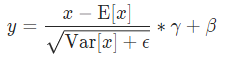# How does batchNorm2d work? (with CoLab code)

Hi,

I organize my problem below.
I hope you utilize this colab code I made.

# 1. Implement new Batchnorm class

I implemented a new BatchNorm2d that is mathematically identical to the original one but has a different coding style.

``````class BatchNorm2d_new(nn.BatchNorm2d):
def forward(self, input):
self._check_input_dim(input)

statistics_tbn = {'mean':input.mean(dim=[0, 2, 3]).detach().clone(), 'var':input.var(dim=[0, 2, 3]).detach().clone()}
statistics_tbn['inv_std'] = torch.reciprocal(torch.sqrt((statistics_tbn['var']+self.eps)))

output = input -  _unsqueeze_(statistics_tbn['mean'])
output = output * _unsqueeze_(statistics_tbn['inv_std'])
output = output * _unsqueeze_(self.weight)
output = output + _unsqueeze_(self.bias)
return output

def _unsqueeze_(tensor):
return tensor.unsqueeze(0).unsqueeze(-1).unsqueeze(-1)

# This function change origin BN to New BN in our network, reclusively.
def convert_bn(module):
module_output = module
if isinstance(module, torch.nn.modules.batchnorm._BatchNorm):
module_output = BatchNorm2d_new(
module.num_features,
module.eps,
module.momentum,
module.affine
)
module_output.weight.data = module.weight.data.clone()
module_output.bias.data = module.bias.data.clone()
module_output.running_mean = module.running_mean
module_output.running_var = module.running_var

for name, child in module.named_children():
name, convert_bn(child),
)
del module
return module_output
``````

# 2. Train on CIFAR10 with origin BN

``````from torchvision.models import resnet18
net = resnet18(pretrained=True)

# Train Resnet18 on CIFAR10
# Results
# accuracy on train dataset (not eval dataset)
[1,     1] loss: 0.007 acc: 0.000
[1,    41] loss: 0.094 acc: 0.500
[1,    81] loss: 0.035 acc: 0.438
[1,   121] loss: 0.029 acc: 0.484
[1,   161] loss: 0.027 acc: 0.484
[1,   201] loss: 0.025 acc: 0.641
[1,   241] loss: 0.024 acc: 0.594
[1,   281] loss: 0.023 acc: 0.719
``````

# 3. Train on CIFAR10 with new BN.

``````from torchvision.models import resnet18
net = resnet18(pretrained=True)
net = convert_bn(net)

# Train Resnet18 on CIFAR10
# Results
# accuracy on train dataset (not eval dataset)
[1,     1] loss: 0.007 acc: 0.000
[1,    41] loss: 0.158 acc: 0.094
[1,    81] loss: 0.052 acc: 0.141
[1,   121] loss: 0.046 acc: 0.172
[1,   161] loss: 0.046 acc: 0.125
[1,   201] loss: 0.045 acc: 0.266
[1,   241] loss: 0.046 acc: 0.094
``````

# 4. Question

As you can see, the accuracy with new BN is too bad then one with original BN. In my opinion, Two BN class should behave the same, since they are mathematically identical. (following torch.batchnorm)Q: Why isn’t learning done with new BN? How should I modify the code?

I hope I explain my question clearly.
Best regards## PS.

1. They trained on train mode (net.train()). I think this is not related to Train & Eval mode. This problem is all about weight(gamma) and bias(beta).
2. Calculating loss and accuracy are implemented in colab code.

I solve a problem after changing my code.

## From

In BatchNorm2d_new class,

``````statistics_tbn = {'mean':input.mean(dim=[0, 2, 3]).detach().clone(), 'var':input.var(dim=[0, 2, 3]).detach().clone()}
statistics_tbn['inv_std'] = torch.reciprocal(torch.sqrt((statistics_tbn['var']+self.eps)))
``````

## To

``````mean = x.mean(dim=[0, 2, 3], keepdim=True)
var = x.var(dim=[0, 2, 3], keepdim=True)
``````

It was because of `detach`. But I still don’t know why `detach` results in non-training.

Using `detach` removes the layer or variable from the model’s training graph, so while the losses are being back propagated the detached outputs do not contribute to the loss, and similarly they are not updated as well. So using `detach` would result in non-training of the layers.

1 Like